Function Repository Resource:

# TransversalHypergraph

Compute the transversal hypergraph of a hypergraph defined by a list of hyperedges and isolated vertices

Contributed by: Daniel McDonald
 ResourceFunction["TransversalHypergraph"][list] computes the transversal hypergraph of list.

## Details and Options

The hypergraph represented by {{v11,v12,},{v21,v22,},,u1,u2,} has vertices v11,v12,,v21,v22,,,u1,u2, and hyperedges {v11,v12,},{v21,v22,},.
Given a hypergraph H having sets V and E of vertices and hyperedges, a transversal T of H is a subset U of V that has a non-empty intersection with each hyperedge in E; a minimal transversal is a transversal containing no other transversal as a proper subset. The transversal hypergraph Tr(H) of H is the hypergraph whose hyperedges are the minimal transversals of H.

## Examples

### Basic Examples (1)

Compute the transversal hypergraph of the hypergraph having vertices a, b, c, d, e, f, g and hyperedges {a,b,c,d}, {b,c}, {c,d,e}:

 In:=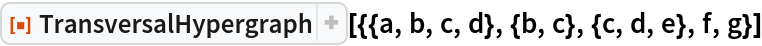Out=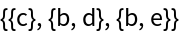### Applications (4)

Define a random hypergraph having n vertices and m hyperedges:

 In:=Out=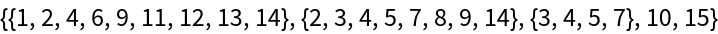Plot the hypergraph:

 In:=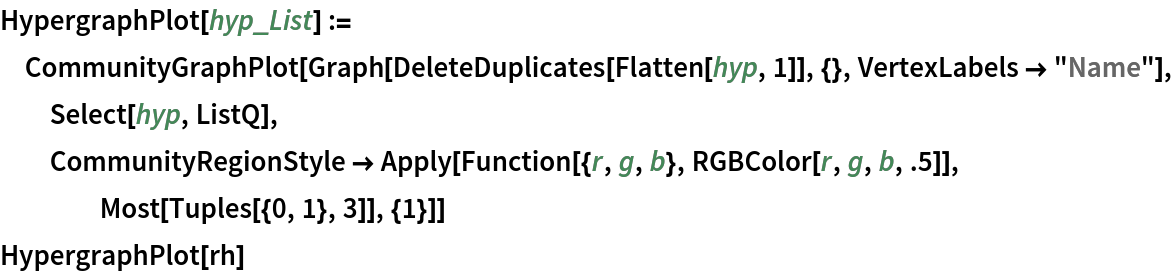Out=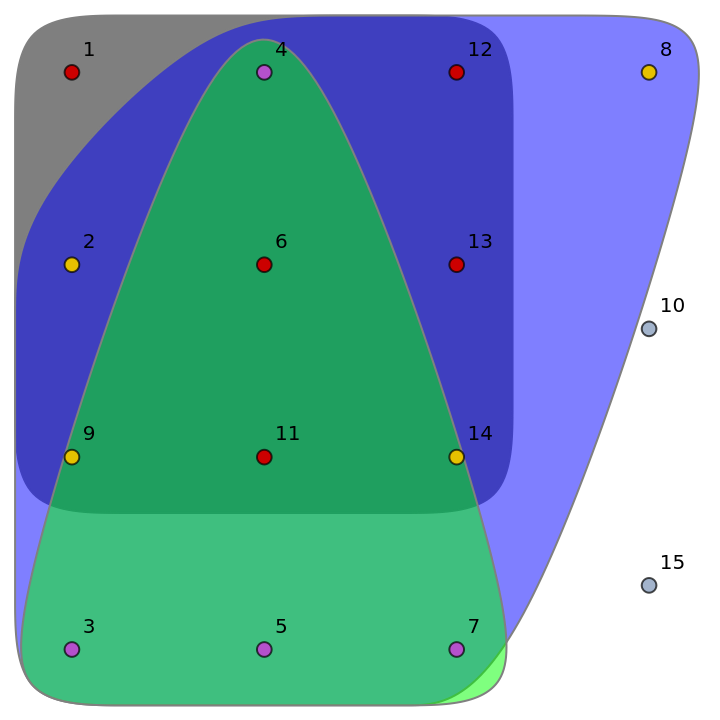Find its transversal hypergraph:

 In:=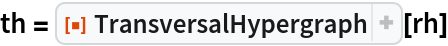Out=Plot the transversal hypergraph:

 In:=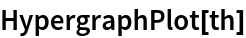Out=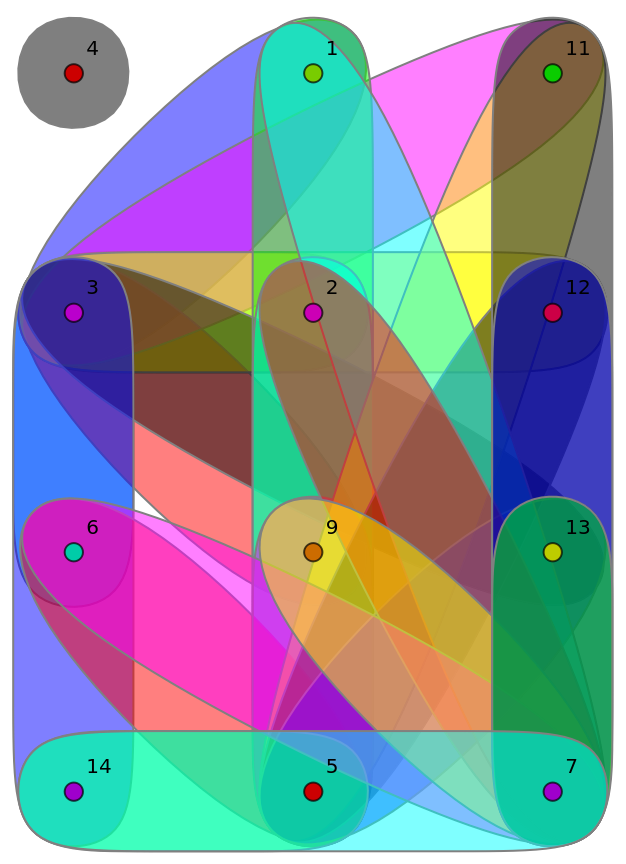Daniel McDonald

## Requirements

Wolfram Language 11.3 (March 2018) or above

## Version History

• 1.0.0 – 16 January 2019